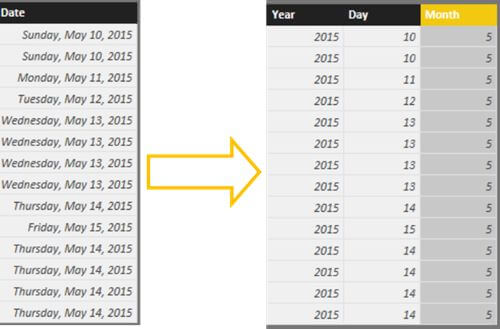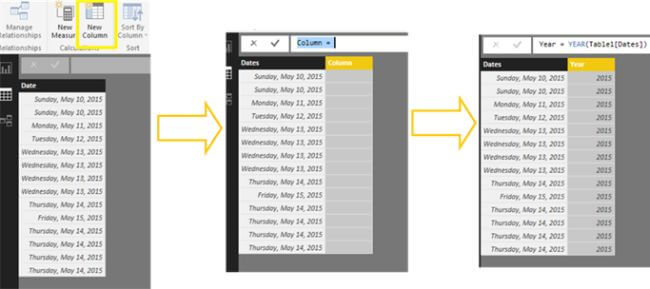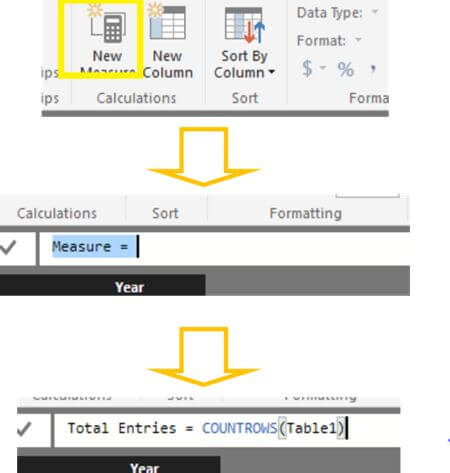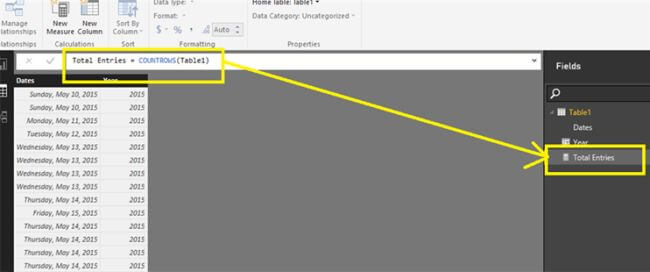## Calculated Column Vs Measures: Power BI

Power BI has the ability to use the given data set and then reuse it to create other calculated fields as required for the reports. In Power BI, there are two ways to create these extra fields. They are:

• Calculated Columns
• Measures

Calculated Columns

Calculated columns are special type of columns that can be added on the given data set. To give an example of a calculated column, let us take an example of a data set where there is a column called Date. Now I would like to extract different values from the given value, for example, the day, month or year.For this, go to the ribbon and click New Column. You can use a DAX formula to define the value of the column. Unlike excel where you can have a different DAX formula for each cell, these calculated columns have a single formula for the whole column. In the following image, I have extracted the year from the dates and added in a new column.Measures

A measure is a numeric calculation used in data analysis. Examples that are commonly found in business reports include sums, averages, minimum or maximum values, counts, or more advanced calculations that you create using a DAX formula.

To create a measure, click on the New Measure icon on the ribbon and use the DAX formula as required. In the given example, I have counted the rows in the given table and saved it as a measure named “Total Entries”.Measures do not show up on the table, but are calculated to give a single value.Calculated Columns Vs Measures

While the ways to create both calculated columns and measures look similar, they have different uses for the purpose of reporting.

Calculated columns must be used when you require a whole column with a specific value in each row. But measures must be used when, there is a single value to be calculated from a given row or a table. For example, the number of rows in the given table.

Calculated Columns acts as a part of the dataset but, measures takes up some additional memory and is calculated every time the data in the dataset changes.